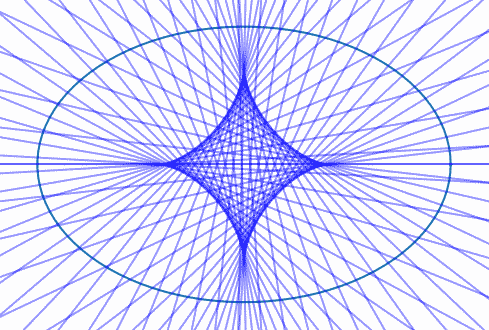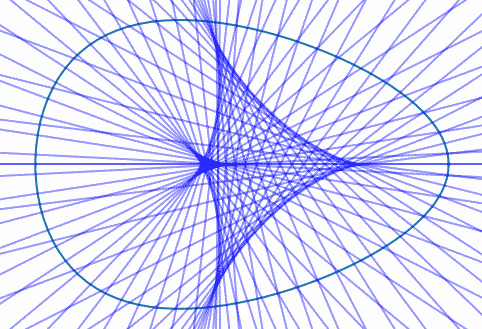# Evolute of an egg

The set of lines perpendicular to a curve are tangent to a second curve called the evolute. The lines perpendicular to the ellipse below are tangent to the curve inside called an astroid.If we replace the ellipse with an egg, we get a similar shape, but less symmetric.The equation for the egg is described here with parameters a = 3, b = 2, and k = 0.1. The ellipse above has the same a and b but k = 0.

I made the lines slightly transparent, setting alpha = 0.4, so the graph would be darker where many lines cross.

Related post: Envelopes of epicycloids